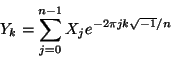Next: , Previous: , Up: What FFTW Really Computes   [Contents][Index]

4.8.1 The 1d Discrete Fourier Transform (DFT)

The forward (FFTW_FORWARD) discrete Fourier transform (DFT) of a 1d complex array X of size n computes an array Y, where:.
The backward (FFTW_BACKWARD) DFT computes:.

FFTW computes an unnormalized transform, in that there is no coefficient in front of the summation in the DFT. In other words, applying the forward and then the backward transform will multiply the input by n.

From above, an FFTW_FORWARD transform corresponds to a sign of -1 in the exponent of the DFT. Note also that we use the standard “in-order” output ordering—the k-th output corresponds to the frequency k/n (or k/T, where T is your total sampling period). For those who like to think in terms of positive and negative frequencies, this means that the positive frequencies are stored in the first half of the output and the negative frequencies are stored in backwards order in the second half of the output. (The frequency -k/n is the same as the frequency (n-k)/n.)